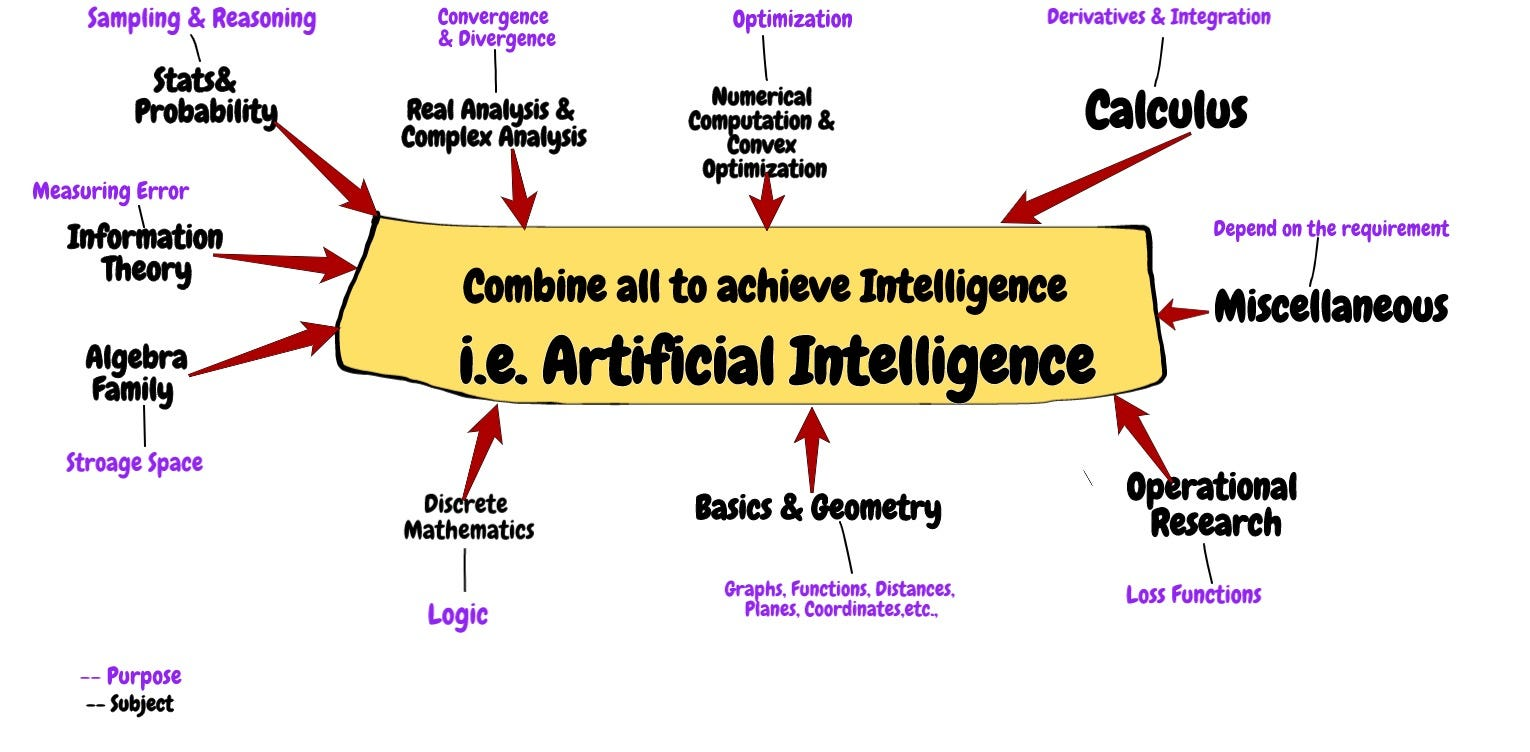# AI & MathematicsAs outline of AI fields can be categorized in the following diagram.

Mathematical Subjects/Concepts will cover in almost all areas (AI-fields) not only specific to Machine Learning and Deep Learning.

How AI-fields and its required Mathematical subjects/concepts involved in algorithms will be covered in the next article briefly.

# AI-Mathematical subjects and required topics

Going through each subject and mention the major concepts required and where and how to use in Algorithms of AI in a short way. By mentioning these reader will be familiar while learning and developing algorithms.

Basic formulas, Functions, Exponential, Logarithms, Euclidean Distance, Plane, Hyperplane, Linear , Non-linear, slope, curves and basics, parabola , circle, etc.,

Introduction: Algebra has multiple variations like Abstract Algebra,Vector Algebra, Linear Algebra.

Abstract Algebra: Laws of Algebra , Groups,homomorphism, Isomorphism, Ring Theory, etc.,

Following are the topics required in Linear Algebra and Vector Algebra. Note that Vector Algebra concepts are few , in some text books they covered in Linear Algebra.

Linear Algebra Concepts: Vectors, Matrices — Types of Matrices(Identity, Inverse,Adjoint) , Tensors, Properties of Matrices (Trace, Determinant, orthogonal,Projections, symmetric, singular ,etc.,), Product Rules- Inner product, Outer product,Vector-Matrix, Matrix Multiplication, Linear Combination of Vectors, Hadamard, Decomposition — Eigen Value Decomposition, SVD, etc., ,Advanced Concepts (uses in QC) — Hilbert Spaces, Tensor product,Hermitian, Unitary, etc.,

You can refresh Linear Algebra in AI & QC, this article will cover almost all topics required in both fields.

Concepts of Vectors applied in ML and Other areas:

Descriptive Statistics: Mean, Variance, Median, Mode, Standard Deviation,Covariance, Expectations, Distributions (Bernoulli, Uniform, Normal (single & multivariate), Poisson, Binomial, Exponential, Gamma), Joint and Marginal Distributions, Probability, axioms of Probability, Conditional Probability, Random Variable,Bayes Rule (Most important) , Chain Rule, Estimation of Parameters: MLE (Maximum Likelihood Estimation), MAP (Maximum A Posterior),Bayesian Networks or Probabilistic Models or Graphical models.

You can see the power of Probability in AI in this article.

Derivatives: Rules of Derivatives: addition, product, division,chain rule, hyperbolic (tanh),applications of derivatives like minima , maxima, etc.,, Integrations (If your using transformations).

Note: We are not using scalar derivatives but these will help in understanding vector and matrix calculus as well as to understand Numerical Computation very well.

Multi variable Calculus, Partial derivatives, Gradient Algorithms.

Variation of Calculus with Linear Algebra: Vector Calculus and Matrix Calculus are most important in Machine Learning and Deep learning

Vector & Matrix Calculus concepts: Gradient , Chain Rule, Jacobians, Hessian.

Following diagram describes Gradient Descent algorithm , it works in Back-propagation (BP) in Neural network architecture for optimizing Parameters.

BP describes Neural Network implementation section.